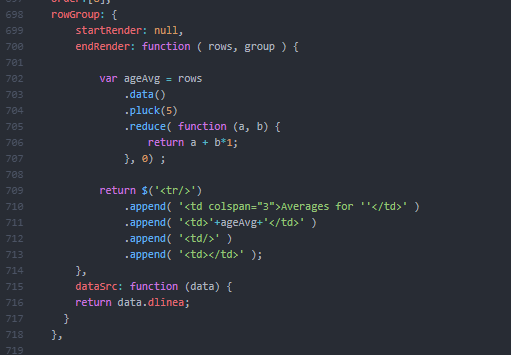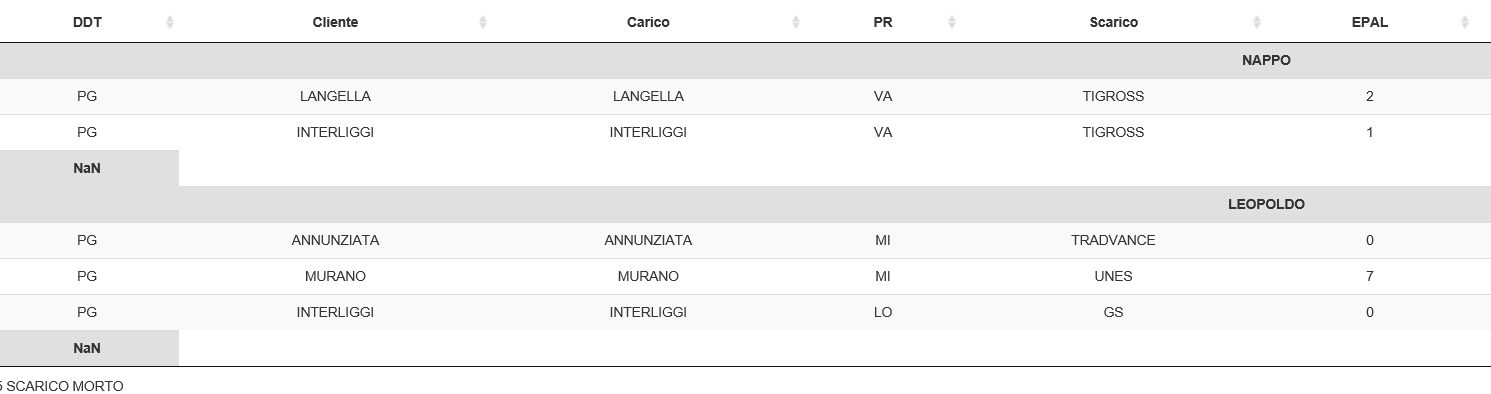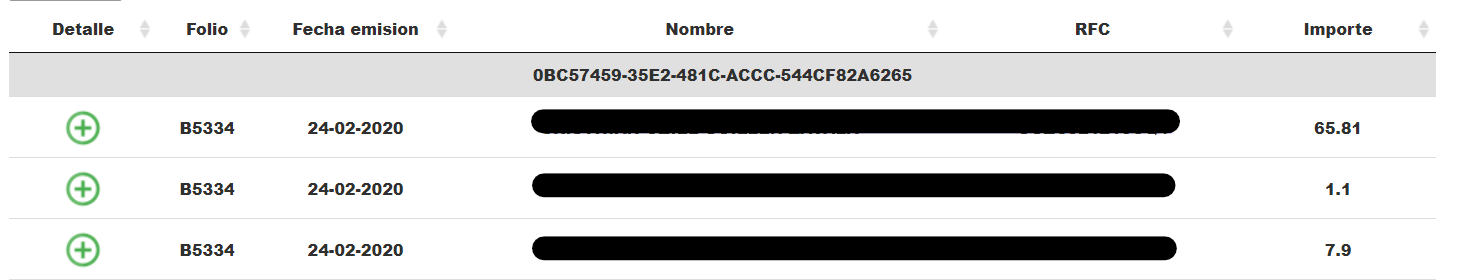# Sum in rowGroup

Hi guys, I shot a few examples and on the form and I did not find the function that in rowGroup makes the sum, can you help me?

• This example shows how to provide averages for each group. You can change the code to shwo the sum by removing `/ rows.count()` on line 20.

Kevin

Hi Kevin,

Look there:

``````var table4 = \$('#dl1').DataTable( {
dom: 'Bfrtip',
ajax: {
url: 'php/table.dl1.php',
type: 'POST'
},
order:,
rowGroup: {
startRender: null,
endRender: function ( rows, group ) {

var ageAvg = rows
.data()
.pluck(5)
.reduce( function (a, b) {
return a + b*1;
}, 0) ;

return \$('<tr/>')
.append( '<td colspan="3">Averages for ''</td>' )
.append( '<td>'+ageAvg+'</td>' )
.append( '<td/>' )
.append( '<td></td>' );
},
dataSrc: function (data) {
return data.dlinea;
}
},

columns:[
{
"data":"ddt"
},
{
"data": "cliente"
},
{
"data": "carico"
},
{
"data":"pr"
},

{
data:null, render: function (data,type,row)
{
return data.scarico+ ' ' +data.scarico_m;
}
},
{
"data": "epal",
},
{
"data": "perd",
},
{
"data":"ind"
},
{
"data": "dlinea"
},
{
"data": "tlinea"
}
],
``````

Return me an error:
in line 710:The error is :

Uncaught SyntaxError: missing ) after argument list

.append( '<td colspan="3">Averages for ''</td>' )

You have two `'` together (`''</td>'`)that is causing the syntax error. Remove one of them.

Kevin

edited April 2

@kthorngren the sum return NaN..

the code js:

``````    rowGroup: {
endRender: function ( rows, group ) {

var ageAvg = rows
.data()
.pluck(5)
.reduce( function (a, b) {
return a + b;
}, 0) ;

return \$('<tr/>')

.append( '<td>'+ageAvg+'</td>' );

},
dataSrc: function (data) {
return data.dlinea;
}
},
``````

The output:• .pluck(5)

Read the `pluck()` docs. This is the data you want to pluck from the row data. You are using objects so change the `5` to the data object you want to some.

If you still get NaN then either provide a test case showing the issue or use console.log statements to debug what is happening in the function.

Kevin

Is the column you want to sum `"data": "epal",`? If so then change pluck to `pluck('epal')`.

Kevin

edited April 2

@kthorngren if i want stamp the sum of epal and perd how i do?

@kthorngren i solved in this way

``````            var epalcount = rows
.data()
.pluck('epal')
.reduce( function (a, b) {
return intVal(a) + intVal(b);
}, 0) ;

var indcount = rows
.data()
.pluck('ind')
.reduce( function (a, b) {
return intVal(a) + intVal(b);
}, 0) ;

var perdcount = rows
.data()
.pluck('perd')
.reduce( function (a, b) {
return intVal(a) + intVal(b);
}, 0) ;

var epcount = (epalcount + perdcount);
``````

i'm so happy!!!! Thanks man for all ur support!!

• Good, I'm glad you got it worked out!

Kevin

• Hello! @antoniocib
I have the same problem, but I just want to add only one column (amount)
I tried it with the examples above but it still doesn't workthis is my code,(copy it from above and adjust it to mine)
rowGroup: {
var sumimporte = rows
.data()
.pluck('importe')
.reduce( function (a, b) {
return intVal(a) + intVal(b);
}, 0) ;
dataSrc: 'uuid'
},
sorry i'm very new to the subject, thanks